Start this free course now. Just create an account and sign in. Enrol and complete the course for a free statement of participation or digital badge if available.

Free course

# 3.3 The five-figure summary and boxplots

As well as giving us a new measure of spread – the interquartile range – the quartiles are important figures in themselves. Our-shaped diagram, Figure 19, gives five important points which help to summarise the shape of a distribution: the median, the two quartiles and the two extremes.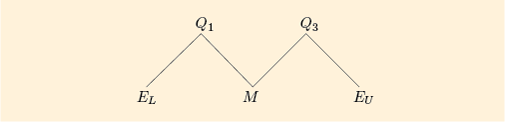Figure 19 Values in a five-figure summary

These are conveniently displayed in the following form, called the five-figure summary of the batch.

## Five-figure summary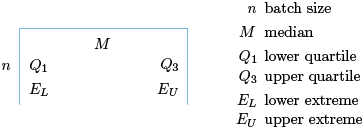Figure 20

## Example 18 Five-figure summary for television price data

For the television price data, we have,,,,and. (You last saw these data in Figure 16, Subsection 3.2.)

Therefore, the five-figure summary of this batch is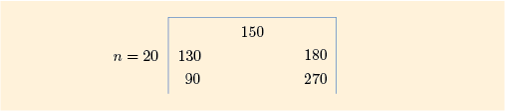Figure 21

This diagram contains the following information about the batch of prices.

• The general level of prices, as measured by the median, is £150.

• The individual prices vary from £90 to £270.

• About 25% of the prices were less than £130.

• About 25% of the prices were more than £180.

• About 50% of the prices were between £130 and £180.

We hope you agree that the five-figure summary is quite an efficient way of presenting a summary of a batch of data.

The five values in a five-figure summary can be very effectively presented in a special diagram called a boxplot. For the 14 gas prices (Figure 15, Subsection 3.2) the diagram looks like Figure 22.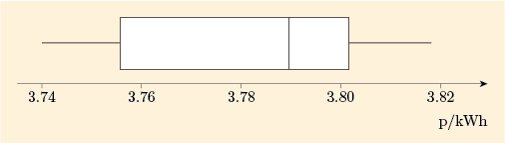Figure 22 Boxplot of batch of 14 gas prices

The central feature of this diagram is a box – hence the name boxplot. The box extends from the lower quartile (at the left-hand edge of the box) to the upper quartile (the right-hand edge). This part of the diagram contains 50% of the values in the batch. The length of this box is thus the interquartile range.

Outside the box are two whiskers. (Boxplots are sometimes called box-and-whisker diagrams.) In many cases, such as in Figure 22, the whiskers extend all the way out to the extremes. Each whisker then covers the end 25% of the batch and the distance between the two whisker-ends is then the range. (You will see examples later where the whiskers do not go right out to the extremes.)

So far we have dealt with four figures from the five-figure summary: the two quartiles and the two extremes. The remaining figure is perhaps the most important: it is the median, whose position is shown by putting a vertical line through the box.

Thus a boxplot shows clearly the division of the data into four parts: the two whiskers and the two sections of the box; these are the four parts of the-shaped diagram and each contains (approximately) 25% of values in the batch (see Figure 21).

## John W. Tukey (1915–2000), inventor of the five-figure summary and boxplot

John Tukey was a prominent and prolific US statistician, based at Princeton University and Bell Laboratories. As well as working in some very technical areas, he was a great promoter of simple ways of picturing and summarising data, and invented both the five-figure summary and the boxplot (except that he called them the ‘five-number summary’ and the ‘box-and-whisker plot’).

He had what has been described as an ‘unusual’ lecturing style. The statistician Peter McCullagh describes a lecture he gave at Imperial College, London in 1977:

Tukey ambled to the podium, a great bear of a man dressed in baggy pants and a black knitted shirt. These might once have been a matching pair, but the vintage was such that it was hard to tell. …The words came …, not many, like overweight parcels, delivered at a slow unfaltering pace. …Tukey turned to face the audience …. ‘Comments, queries, suggestions?’ he asked …. As he waited for a response, he clambered onto the podium and manoeuvred until he was sitting cross-legged facing the audience. …We in the audience sat like spectators at the zoo waiting for the great bear to move or say something. But the great bear appeared to be doing the same thing, and the feeling was not comfortable. …After a long while, …he extracted from his pocket a bag of dried prunes and proceeded to eat them in silence, one by one. The war of nerves continued …four prunes, five prunes. …How many prunes would it take to end the silence?

(Source: McCullagh, P. (2003) ‘John Wilder Tukey’, Biographical Memoirs of Fellows of the Royal Society, vol. 49, pp. 537–55.)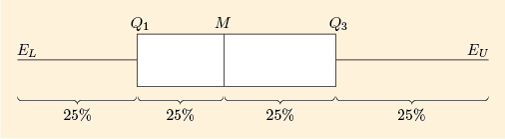Figure 23 A standard boxplot with annotation

A typical boxplot looks something like Figure 23 because in most batches of data the values are more densely packed in the middle of the batch and are less densely packed in the extremes. This means that each whisker is usually longer than half the length of the box. This is illustrated again in the next example.

## Example 19 Boxplot for the prices of small televisions

The boxplot for the batch of 20 television prices (last worked with in Example 18) is shown in Figure 24.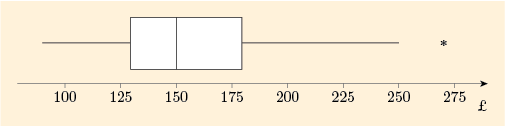Figure 24 Boxplot of batch of 20 television prices

You can see that each whisker is longer than half the length of the box.

However, this boxplot has a new feature. The whisker on the left goes right down to the lower extreme. But the whisker on the right does not go right to the upper extreme. The highest extreme data value, 270, which might potentially be regarded as an outlier, is marked separately with a star. Then the whisker extends only to cover the data values that are not extreme enough to be regarded as potential outliers. The highest of these values is 250.

(This course does not describe the rule to decide which data values (if any) can be regarded as potential outliers that are plotted separately on the diagram. This is another issue that may be dealt with differently by different authors and different software.)

Example 19 is the subject of the following screencast. [Note that the reference to ‘Unit 2’ should be ‘this course’ and ‘Figure 18’ should be ‘Figure 23’. Unit 2 and Figure 18 are references to the Open University course from which this material is adapted.]Skip transcript: Screencast 4 Interpreting a boxplot

#### Transcript: Screencast 4 Interpreting a boxplot

INSTRUCTOR: In this screencast, I’m going to talk about interpreting a boxplot. And what we have here is an example of a boxplot. And it happens to be Figure 18 from Subsection 3.3 of Unit 2. And it’s a boxplot of the small television prices. And you can see here that television prices are given in pounds, and they go from just under £100 up to £275.

The first thing on the boxplot to look at is the box itself – in particular, to look at the ends of the box. The end on the left hand side shows us where the lower quartile is. And here is about £130. The end on the right hand side shows us where the upper quartile is – Q3. And this translates to about £180. So the lower quartile is about £130, and the upper quartile is about £180.

The line in the middle of the box shows us where the median is. And this is about £150. So the price of the median small television is £150. Notice in this example, it is quite clear where the line is in the middle of the box. There are some examples where the median is the same as the lower quartile or where the median is the same as the upper quartile. And then you won’t actually see a line in the box. The line will be at one end or the other.

The other thing to notice on the boxplot are the two whiskers. So there’s a whisker on the right hand side and a whisker on the left hand side. The whisker on the right hand side shows us where the values that are high but not too high are. Similarly, on the left hand side, the whisker on the left hand side shows us where the values are low but not too low are.

And finally, notice there’s one point here marked all by itself. And this is a value that we wonder whether it’s too high. In other words, we’re marking this one out as a potential outlier. This shows all the elements that are on a boxplot. And one thing we can use these elements for is to say something about the symmetry of the data.

And one thing we can look at is where the median is relative to the two ends of the box. So here we notice that the left hand side is short relative to the right hand side. We can look at the whiskers in the same way, and notice that the whisker on the left hand side is relatively short. And the whisker on the right hand side is relatively long.

And both these observations together suggest that the data are right-skew. The data tends to be more spread out on the right hand side of the median relative to the data on the left hand side the median. And notice, in doing this, we haven’t actually taken account of the outlier. If we took the outlier into account as well, this would only emphasise more that the data are right-skew. Because this adds to the impression that the data are more spread out to the right of the median relative to the left of the median.

End transcript: Screencast 4 Interpreting a boxplot
Screencast 4 Interpreting a boxplot
Interactive feature not available in single page view (see it in standard view).

One important use of boxplots is to picture and describe the overall shape of a batch of data.

## Example 20 Skew televisions

The stemplot of small television prices, last seen in Figure 16 (Subsection 3.2), shows a lack of symmetry. Since the higher values are more spread out than the lower values, the data are right-skew.

The boxplot of these data, given in Figure 22, also shows this right-skew fairly clearly. In the box, the right-hand part (corresponding to higher prices) is rather longer than the left-hand part, and the right-hand whisker is longer than the left-hand whisker.

## Activity 13 Skew gas prices?

A stemplot of the gas price data from Activity 2 (Subsection 1.2) is shown, yet again, in Figure 25.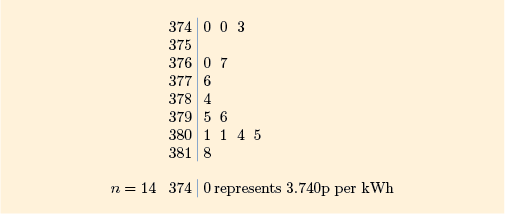Figure 25 Stemplot of 14 gas prices

(a) Prepare a five-figure summary of the batch.

### Discussion

All the necessary figures have already been calculated. You found the median (3.790) in Activity 2 and the quartiles (,) in Activity 10. The extremes (,) and the batch size () are clearly shown in the stemplot.

So the five-figure summary is as follows: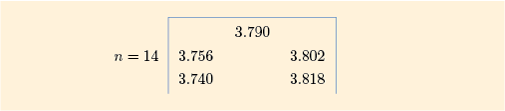Figure 26

(b) Figure 27 shows the boxplot of these data that you have already seen in Figure 22. What do the stemplot and boxplot tell us about the symmetry and/or skewness of the batch?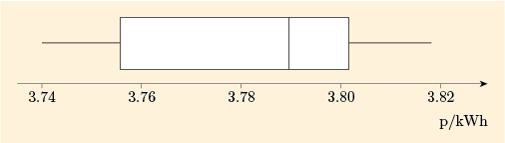Figure 27 Boxplot of batch of 14 gas prices

### Discussion

Looking at the stemplot, on the whole the lower values are more spread out, indicating that the data are not symmetric and are left-skew.

The central box of the boxplot again shows left skewness, with the left-hand part of the box being clearly longer than the right-hand part. However, this skewness does not show up in the lengths of the whiskers in this batch – they are both the same length.

## Example 21 Camera prices: skew or not?

In Example 20 and Activity 13 you saw how boxplots look for batches of data that are right-skew or left-skew. What happens in a batch that is more symmetrical?

For the small batch of camera prices from Table 2 (Subsection 1.2), a (stretched) stemplot is shown in Figure 28.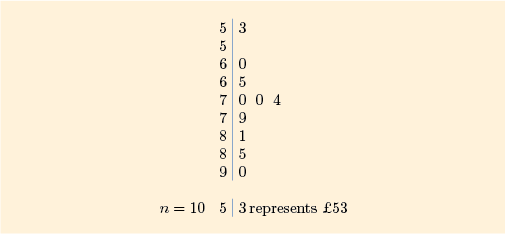Figure 28 Stemplot of ten camera prices

The stemplot looks reasonably symmetric.

A boxplot of the data, Figure 29, confirms the impression of symmetry. The two parts of the box are roughly equal in length, and the two whiskers are also roughly equal in length.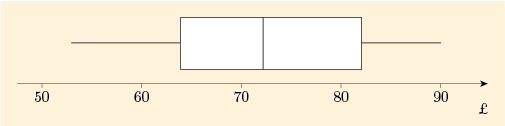Figure 29 Boxplot of batch of ten camera prices

You have now spent quite a lot of time looking at various ways of investigating prices and, in particular, at methods of measuring the location and spread of the prices of particular commodities.

In order to begin to answer our question, Are people getting better or worse off?, we need to know not just location (and spread) of prices but also how these prices are changing from year to year. That is the subject of the rest of this course.

M140_1